Test: Measurement of Power & Energy - 1

# Test: Measurement of Power & Energy - 1 - Electrical Engineering (EE)

Test Description

## 10 Questions MCQ Test GATE Electrical Engineering (EE) 2024 Mock Test Series - Test: Measurement of Power & Energy - 1

Test: Measurement of Power & Energy - 1 for Electrical Engineering (EE) 2023 is part of GATE Electrical Engineering (EE) 2024 Mock Test Series preparation. The Test: Measurement of Power & Energy - 1 questions and answers have been prepared according to the Electrical Engineering (EE) exam syllabus.The Test: Measurement of Power & Energy - 1 MCQs are made for Electrical Engineering (EE) 2023 Exam. Find important definitions, questions, notes, meanings, examples, exercises, MCQs and online tests for Test: Measurement of Power & Energy - 1 below.
Solutions of Test: Measurement of Power & Energy - 1 questions in English are available as part of our GATE Electrical Engineering (EE) 2024 Mock Test Series for Electrical Engineering (EE) & Test: Measurement of Power & Energy - 1 solutions in Hindi for GATE Electrical Engineering (EE) 2024 Mock Test Series course. Download more important topics, notes, lectures and mock test series for Electrical Engineering (EE) Exam by signing up for free. Attempt Test: Measurement of Power & Energy - 1 | 10 questions in 30 minutes | Mock test for Electrical Engineering (EE) preparation | Free important questions MCQ to study GATE Electrical Engineering (EE) 2024 Mock Test Series for Electrical Engineering (EE) Exam | Download free PDF with solutions
 1 Crore+ students have signed up on EduRev. Have you?
Test: Measurement of Power & Energy - 1 - Question 1

### Which one of the following meters is an induction type instrument?

Detailed Solution for Test: Measurement of Power & Energy - 1 - Question 1

An energymeter is an induction and an integrating type instrument.

Test: Measurement of Power & Energy - 1 - Question 2

### The error caused by pressure coil inductance in

Detailed Solution for Test: Measurement of Power & Energy - 1 - Question 2

To reduce the error in the wattmeter reading due to pressure coil inductance, the wattmeters are compensated by connecting a capacitor in parallel with a portion of series resistance (multiplier) as shown below.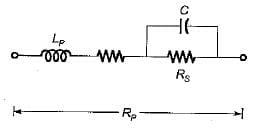Test: Measurement of Power & Energy - 1 - Question 3

### Power at RF is measured using

Detailed Solution for Test: Measurement of Power & Energy - 1 - Question 3

At AF (audiofrequency) and RF (radio frequency) it is easier to measure voltage, current and impedance, than to measure- power. Direct measurement of power is not done in this range. Here, power is calculated from the equation
P = E2IR or P = I2 R

Test: Measurement of Power & Energy - 1 - Question 4

Creeping in a single phase induction type energy meter may be due to

Test: Measurement of Power & Energy - 1 - Question 5

In a single phase induction type energy meter, the lag adjustment is done

Detailed Solution for Test: Measurement of Power & Energy - 1 - Question 5

For the energy meter to record true energy the pressure coil should be highly inductive (i.e, Δ = 90°). Thus, for the angle between the shunt magnet flux (φρ) and the supply voltage to be 90°, a lagging coil is placed between the potential coil and the rotating aluminium disc. Lag adjustment sometimes is called power factor or quadrature or inductive load adjustment.

Test: Measurement of Power & Energy - 1 - Question 6

A wattmeter has a current coil of 0.1 Ω resistance and a pressure coil of 6500 Ω respectively. The wattmeter shows a reading of 12 A at 250 V with unity power factor. The percentage errors, due to resistance only with each of the two methods of connections shown below will be respectively

Detailed Solution for Test: Measurement of Power & Energy - 1 - Question 6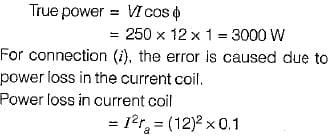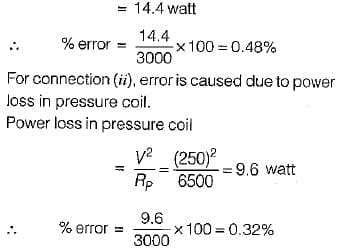Test: Measurement of Power & Energy - 1 - Question 7

The power factor in a 3-phase circuit using two wattmeter method is given by

Detailed Solution for Test: Measurement of Power & Energy - 1 - Question 7

From two-wattmeter method of measurement of power, we have: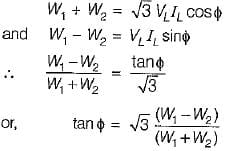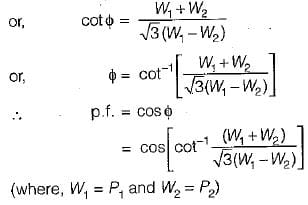Test: Measurement of Power & Energy - 1 - Question 8

Phantom leading for testing of energy meter is used

Detailed Solution for Test: Measurement of Power & Energy - 1 - Question 8

When the energy meter is tested under high current rating, there is lot of waste of power. To reduce this unwanted power loss, Phantom or Fictitious loading is done.

Test: Measurement of Power & Energy - 1 - Question 9

The braking torque provided by a permanent magnet in a single phase meter is proportional to the

Detailed Solution for Test: Measurement of Power & Energy - 1 - Question 9

Braking torque provided by the permanent magnet is given by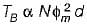Where,
N = speed of the disc in rpm
φm = maximum value of flux of the permanent magnet
d = distance of the permanent magnet from the centre of the revolving disc

Test: Measurement of Power & Energy - 1 - Question 10

The meter constant of a 230 V, 10 A watthour meter is 1800 revolutions per kWh. The meter is tested at half load and rated voltage and unity power factor. The meter is found to make 80 rev. in 138 seconds. The meter error at half load is

Detailed Solution for Test: Measurement of Power & Energy - 1 - Question 10

Given, energy meter constant,
K = 1800 revolutions per kWh Actuai energy or true energy consumed by the energy meter at half load during 138 seconds is: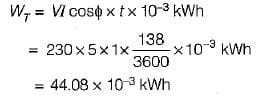Also, measured energy or energy recorded is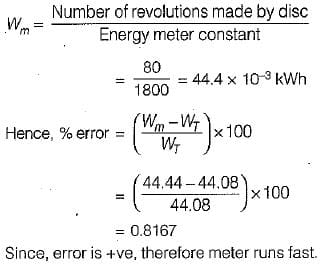## GATE Electrical Engineering (EE) 2024 Mock Test Series

23 docs|285 tests
Information about Test: Measurement of Power & Energy - 1 Page
In this test you can find the Exam questions for Test: Measurement of Power & Energy - 1 solved & explained in the simplest way possible. Besides giving Questions and answers for Test: Measurement of Power & Energy - 1, EduRev gives you an ample number of Online tests for practice

## GATE Electrical Engineering (EE) 2024 Mock Test Series

23 docs|285 tests